# 225 Results

View
Selected filters:
• Algebra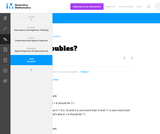Unrestricted Use
CC BY
Rating

This is a task from the Illustrative Mathematics website that is one part of a complete illustration of the standard to which it is aligned. Each task has at least one solution and some commentary that addresses important aspects of the task and its potential use.

Subject:
Algebra
Material Type:
Activity/Lab
Provider:
Illustrative Mathematics
Provider Set:
Illustrative Mathematics
Author:
Illustrative Mathematics
02/16/2018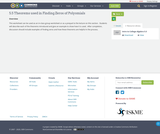Unrestricted Use
CC BY
Rating

This worksheet can be used as an in-class group worksheet or as a prequel to the lecture on this section. Students will describe each of the theorems introduced and give an example to show how it is used. After completion, discussion should include examples of finding zeros and how these theorems are helpful in the process.

Subject:
Mathematics
Algebra
Material Type:
Lesson Plan
Author:
Sandy Porter
08/03/2020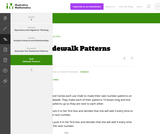Unrestricted Use
CC BY
Rating

This is a task from the Illustrative Mathematics website that is one part of a complete illustration of the standard to which it is aligned. Each task has at least one solution and some commentary that addresses important aspects of the task and its potential use.

Subject:
Algebra
Material Type:
Activity/Lab
Provider:
Illustrative Mathematics
Provider Set:
Illustrative Mathematics
Author:
Illustrative Mathematics
02/16/2018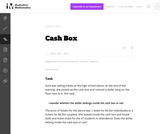Unrestricted Use
CC BY
Rating

The given solutions for this task involve the creation and solving of a system of two equations and two unknowns, with the caveat that the context of the problem implies that we are interested only in non-negative integer solutions. Indeed, in the first solution, we must also restrict our attention to the case that one of the variables is further even.

Subject:
Mathematics
Algebra
Material Type:
Activity/Lab
Provider:
Illustrative Mathematics
Provider Set:
Illustrative Mathematics
Author:
Illustrative Mathematics
01/18/2013Unrestricted Use
CC BY
Rating

This task examines the ways in which the plane can be covered by regular polygons in a very strict arrangement called a regular tessellation. These tessellations are studied here using algebra, which enters the picture via the formula for the measure of the interior angles of a regular polygon (which should therefore be introduced or reviewed before beginning the task). The goal of the task is to use algebra in order to understand which tessellations of the plane with regular polygons are possible.

Subject:
Mathematics
Algebra
Geometry
Material Type:
Activity/Lab
Provider:
Illustrative Mathematics
Provider Set:
Illustrative Mathematics
Author:
Illustrative Mathematics
01/21/2013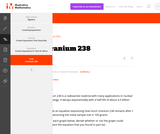Unrestricted Use
CC BY
Rating

This is a task from the Illustrative Mathematics website that is one part of a complete illustration of the standard to which it is aligned. Each task has at least one solution and some commentary that addresses important aspects of the task and its potential use.

Subject:
Algebra
Material Type:
Activity/Lab
Provider:
Illustrative Mathematics
Provider Set:
Illustrative Mathematics
Author:
Illustrative Mathematics
02/16/2018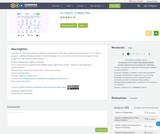Conditional Remix & Share Permitted
CC BY-NC-SA
Rating

A+ Click is an interactive collection of more than 3700 math problems and answers for K-1 K-12 school program. It defines the personal level of math knowledge. You move up into the next level if you give 5 correct answers in a row. Practice makes perfect.

Subject:
Mathematics
Algebra
Geometry
Numbers and Operations
Material Type:
Activity/Lab
Assessment
Game
Lecture Notes
Author:
Igor Kokcharov
08/04/2020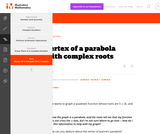Unrestricted Use
CC BY
Rating

This is a task from the Illustrative Mathematics website that is one part of a complete illustration of the standard to which it is aligned. Each task has at least one solution and some commentary that addresses important aspects of the task and its potential use.

Subject:
Algebra
Material Type:
Activity/Lab
Provider:
Illustrative Mathematics
Provider Set:
Illustrative Mathematics
Author:
Illustrative Mathematics
02/16/2018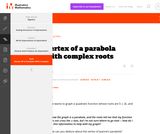Unrestricted Use
CC BY
Rating

This is a task from the Illustrative Mathematics website that is one part of a complete illustration of the standard to which it is aligned. Each task has at least one solution and some commentary that addresses important aspects of the task and its potential use.

Subject:
Algebra
Material Type:
Activity/Lab
Provider:
Illustrative Mathematics
Provider Set:
Illustrative Mathematics
Author:
Illustrative Mathematics
02/16/2018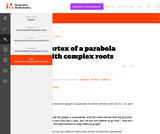Unrestricted Use
CC BY
Rating

This is a task from the Illustrative Mathematics website that is one part of a complete illustration of the standard to which it is aligned. Each task has at least one solution and some commentary that addresses important aspects of the task and its potential use.

Subject:
Algebra
Material Type:
Activity/Lab
Provider:
Illustrative Mathematics
Provider Set:
Illustrative Mathematics
Author:
Illustrative Mathematics
02/16/2018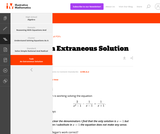Unrestricted Use
CC BY
Rating

This is a task from the Illustrative Mathematics website that is one part of a complete illustration of the standard to which it is aligned. Each task has at least one solution and some commentary that addresses important aspects of the task and its potential use.

Subject:
Algebra
Material Type:
Activity/Lab
Provider:
Illustrative Mathematics
Provider Set:
Illustrative Mathematics
Author:
Illustrative Mathematics
02/16/2018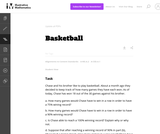Unrestricted Use
CC BY
Rating

This task provides a simple but interesting and realistic context in which students are led to set up a rational equation (and a rational inequality) in one variable, and then solve that equation/inequality for an unknown variable.

Subject:
Mathematics
Algebra
Material Type:
Activity/Lab
Provider:
Illustrative Mathematics
Provider Set:
Illustrative Mathematics
Author:
Illustrative Mathematics
12/15/2012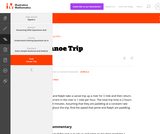Unrestricted Use
CC BY
Rating

This is a task from the Illustrative Mathematics website that is one part of a complete illustration of the standard to which it is aligned. Each task has at least one solution and some commentary that addresses important aspects of the task and its potential use.

Subject:
Algebra
Material Type:
Activity/Lab
Provider:
Illustrative Mathematics
Provider Set:
Illustrative Mathematics
Author:
Illustrative Mathematics
02/16/2018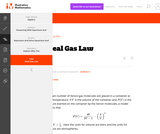Unrestricted Use
CC BY
Rating

This is a task from the Illustrative Mathematics website that is one part of a complete illustration of the standard to which it is aligned. Each task has at least one solution and some commentary that addresses important aspects of the task and its potential use.

Subject:
Algebra
Material Type:
Activity/Lab
Provider:
Illustrative Mathematics
Provider Set:
Illustrative Mathematics
Author:
Illustrative Mathematics
02/16/2018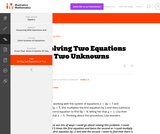Unrestricted Use
CC BY
Rating

This is a task from the Illustrative Mathematics website that is one part of a complete illustration of the standard to which it is aligned. Each task has at least one solution and some commentary that addresses important aspects of the task and its potential use.

Subject:
Algebra
Material Type:
Activity/Lab
Provider:
Illustrative Mathematics
Provider Set:
Illustrative Mathematics
Author:
Illustrative Mathematics
02/16/2018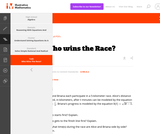Unrestricted Use
CC BY
Rating

This is a task from the Illustrative Mathematics website that is one part of a complete illustration of the standard to which it is aligned. Each task has at least one solution and some commentary that addresses important aspects of the task and its potential use.

Subject:
Algebra
Material Type:
Activity/Lab
Provider:
Illustrative Mathematics
Provider Set:
Illustrative Mathematics
Author:
Illustrative Mathematics
02/16/2018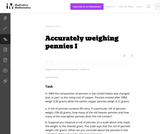Unrestricted Use
CC BY
Rating

This problem involves solving a system of algebraic equations from a context: depending how the problem is interpreted, there may be one equation or two.

Subject:
Mathematics
Algebra
Material Type:
Activity/Lab
Provider:
Illustrative Mathematics
Provider Set:
Illustrative Mathematics
Author:
Illustrative Mathematics
08/15/2012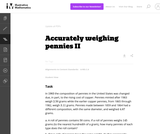Unrestricted Use
CC BY
Rating

This task is a somewhat more complicated version of "Accurately weighing pennies I'' as a third equation is needed in order to solve part (a) explicitly. Instead, students have to combine the algebraic techniques with some additional problem-solving (numerical reasoning, informed guess-and-check, etc.)

Subject:
Mathematics
Algebra
Material Type:
Activity/Lab
Provider:
Illustrative Mathematics
Provider Set:
Illustrative Mathematics
Author:
Illustrative Mathematics
08/15/2012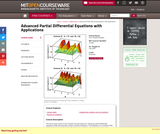Conditional Remix & Share Permitted
CC BY-NC-SA
Rating

" The focus of the course is the concepts and techniques for solving the partial differential equations (PDE) that permeate various scientific disciplines. The emphasis is on nonlinear PDE. Applications include problems from fluid dynamics, electrical and mechanical engineering, materials science, quantum mechanics, etc."

Subject:
Algebra
Material Type:
Full Course
Provider:
MIT
Provider Set:
MIT OpenCourseWare
Author:
Rosales, Rodolfo R.
01/01/2009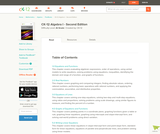Conditional Remix & Share Permitted
CC BY-NC-SA
Rating

A work in progress, CK-12's Algebra I Second Edition is a clear presentation of algebra for the high school student. Topics include: Equations and Functions, Real Numbers, Equations of Lines, Solving Systems of Equations and Quadratic Equations.

Subject:
Algebra
Functions
Material Type:
Textbook
Provider:
CK-12 Foundation
Provider Set:
CK-12 FlexBook
Author:
Andrew
Annamaria
Anne
Eve
Farbizio
Gloag
Rawley
12/03/2010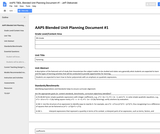Conditional Remix & Share Permitted
CC BY-NC
Rating

Students are expected to learn how to factor polynomials with an emphasis on quadratic expressions.

Subject:
Mathematics
Algebra
Material Type:
Unit of Study
Provider:
Michigan Virtual
Author:
Jeff Oleksinski
04/11/2017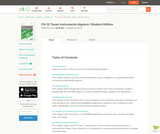Conditional Remix & Share Permitted
CC BY-NC-SA
Rating

CK-12's Texas Instruments Algebra I Student Edition Flexbook allows students to better utilize a graphing calculator in understanding the fundamental concepts of algebra.

Subject:
Algebra
Material Type:
Textbook
Provider:
CK-12 Foundation
Provider Set:
CK-12 FlexBook
Author:
Jordan, Lori
12/08/2010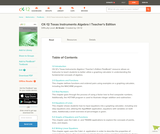Conditional Remix & Share Permitted
CC BY-NC-SA
Rating

CK-12's Texas Instruments Algebra I Teacher's Edition Flexbook allows an Instructor to teach students to better utilize a graphing calculator in understanding the fundamental concepts of algebra.

Subject:
Algebra
Material Type:
Textbook
Provider:
CK-12 Foundation
Provider Set:
CK-12 FlexBook
Author:
Jordan, Lori
12/13/2010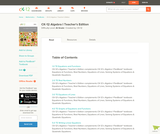Conditional Remix & Share Permitted
CC BY-NC-SA
Rating

CK-12 Foundation's Algebra I Teacher's Edition FlexBook complements CK-12's Algebra I Student Edition. The solution and assessment guides are available upon request.

Subject:
Algebra
Material Type:
Textbook
Provider:
CK-12 Foundation
Provider Set:
CK-12 FlexBook
Author:
Fay-Zenk, Mary
McFarland, Andrew
11/06/2009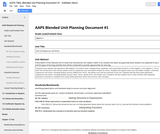Conditional Remix & Share Permitted
CC BY-NC-SA
Rating

Students have already had experience with patterns of numbers when studying linear, quadratic, and exponential functions in both Algebra 1 and Algebra 2. This is their first introduction to the formal notation used for sequences and summation notation for series. Students will explore series and summation notation. Arithmetic sequences and series, including finding common differences, terms, and partial sums will be examined in this unit. Students will also learn about geometric sequences and series, including finding common ratios, terms, partial sums, and infinite sums. Students will also explore how to write numbers with repeating decimals as fractions in simplest form using infinite geometric series and common ratio concepts.

Subject:
Mathematics
Algebra
Material Type:
Unit of Study
Provider:
Michigan Virtual
Author:
Kathleen Ilaoro
03/24/2017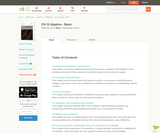Conditional Remix & Share Permitted
CC BY-NC-SA
Rating

CK-12 Foundation's Basic Algebra FlexBook is an introduction to the algebraic topics of functions, equations, and graphs for middle-school and high-school students.

Subject:
Algebra
Functions
Material Type:
Textbook
Provider:
CK-12 Foundation
Provider Set:
CK-12 FlexBook
Author:
Farbizio, Annamaria
Gloag, Andrew
Gloag, Anne
Kramer, Melissa
09/21/2010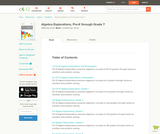Rating

CK-12 Algebra Explorations is a hands-on series of activities that guides students from Pre-K to Grade 7 through algebraic concepts.

Subject:
Algebra
Functions
Material Type:
Activity/Lab
Lesson Plan
Textbook
Provider:
CK-12 Foundation
Provider Set:
CK-12 FlexBook
Author:
Mary Cavanagh, Carol Findell, Carole Greenes
10/04/2011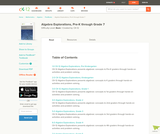Conditional Remix & Share Permitted
CC BY-NC-SA
Rating

CK-12 Algebra Explorations is a hands-on series of activities that guides students from Pre-K to Grade 7 through algebraic concepts.

Subject:
Algebra
Material Type:
Activity/Lab
Teaching/Learning Strategy
Provider:
CK-12 Foundation
Provider Set:
CK-12 FlexBook
Author:
Carol ­ Findell, Mary ­Cavanagh, Carole ­Greenes
02/23/2012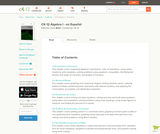Conditional Remix & Share Permitted
CC BY-NC-SA
Rating

CK-12 Foundation's Algebra FlexBook is an introduction to algebraic concepts for the high school student. Topics include: Equations & Functions, Real Numbers, Equations of Lines, Solving Systems of Equations & Quadratic Equations.

Subject:
Algebra
Material Type:
Textbook
Provider:
CK-12 Foundation
Provider Set:
CK-12 FlexBook
Author:
Gloag, Andrew
Gloag, Anne
11/23/2010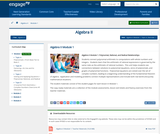Conditional Remix & Share Permitted
CC BY-NC-SA
Rating

Students connect polynomial arithmetic to computations with whole numbers and integers.  Students learn that the arithmetic of rational expressions is governed by the same rules as the arithmetic of rational numbers.  This unit helps students see connections between solutions to polynomial equations, zeros of polynomials, and graphs of polynomial functions.  Polynomial equations are solved over the set of complex numbers, leading to a beginning understanding of the fundamental theorem of algebra.  Application and modeling problems connect multiple representations and include both real world and purely mathematical situations.

Subject:
Algebra
Material Type:
Module
Provider:
New York State Education Department
Provider Set:
EngageNY
05/14/2013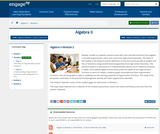Conditional Remix & Share Permitted
CC BY-NC-SA
Rating

Module 2 builds on students previous work with units and with functions from Algebra I, and with trigonometric ratios and circles from high school Geometry. The heart of the module is the study of precise definitions of sine and cosine (as well as tangent and the co-functions) using transformational geometry from high school Geometry. This precision leads to a discussion of a mathematically natural unit of rotational measure, a radian, and students begin to build fluency with the values of the trigonometric functions in terms of radians. Students graph sinusoidal and other trigonometric functions, and use the graphs to help in modeling and discovering properties of trigonometric functions. The study of the properties culminates in the proof of the Pythagorean identity and other trigonometric identities.

Subject:
Algebra
Material Type:
Module
Provider:
New York State Education Department
Provider Set:
EngageNY
08/15/2014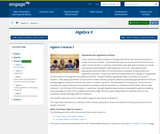Conditional Remix & Share Permitted
CC BY-NC-SA
Rating

In this module, students synthesize and generalize what they have learned about a variety of function families.  They extend the domain of exponential functions to the entire real line (N-RN.A.1) and then extend their work with these functions to include solving exponential equations with logarithms (F-LE.A.4).  They explore (with appropriate tools) the effects of transformations on graphs of exponential and logarithmic functions.  They notice that the transformations on a graph of a logarithmic function relate to the logarithmic properties (F-BF.B.3).  Students identify appropriate types of functions to model a situation.  They adjust parameters to improve the model, and they compare models by analyzing appropriateness of fit and making judgments about the domain over which a model is a good fit.  The description of modeling as, the process of choosing and using mathematics and statistics to analyze empirical situations, to understand them better, and to make decisions, is at the heart of this module.  In particular, through repeated opportunities in working through the modeling cycle (see page 61 of the CCLS), students acquire the insight that the same mathematical or statistical structure can sometimes model seemingly different situations.

Subject:
Algebra
Material Type:
Module
Provider:
New York State Education Department
Provider Set:
EngageNY
09/16/2014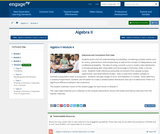Conditional Remix & Share Permitted
CC BY-NC-SA
Rating

Students build a formal understanding of probability, considering complex events such as unions, intersections, and complements as well as the concept of independence and conditional probability.  The idea of using a smooth curve to model a data distribution is introduced along with using tables and techonolgy to find areas under a normal curve.  Students make inferences and justify conclusions from sample surveys, experiments, and observational studies.  Data is used from random samples to estimate a population mean or proportion.  Students calculate margin of error and interpret it in context.  Given data from a statistical experiment, students use simulation to create a randomization distribution and use it to determine if there is a significant difference between two treatments.

Subject:
Algebra
Material Type:
Module
Provider:
New York State Education Department
Provider Set:
EngageNY
03/24/2016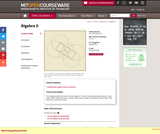Conditional Remix & Share Permitted
CC BY-NC-SA
Rating

This undergraduate level course follows Algebra I. Topics include group representations, rings, ideals, fields, polynomial rings, modules, factorization, integers in quadratic number fields, field extensions, and Galois theory.

Subject:
Algebra
Material Type:
Full Course
Provider:
MIT
Provider Set:
MIT OpenCourseWare
Author:
Artin, Michael
01/01/2011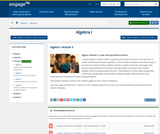Conditional Remix & Share Permitted
CC BY-NC-SA
Rating

In earlier grades, students define, evaluate, and compare functions and use them to model relationships between quantities. In this module, students extend their study of functions to include function notation and the concepts of domain and range. They explore many examples of functions and their graphs, focusing on the contrast between linear and exponential functions. They interpret functions given graphically, numerically, symbolically, and verbally; translate between representations; and understand the limitations of various representations.

Subject:
Algebra
Material Type:
Module
Provider:
New York State Education Department
Provider Set:
EngageNY
09/17/2013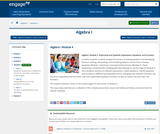Conditional Remix & Share Permitted
CC BY-NC-SA
Rating

In earlier modules, students analyze the process of solving equations and developing fluency in writing, interpreting, and translating between various forms of linear equations (Module 1) and linear and exponential functions (Module 3). These experiences combined with modeling with data (Module 2), set the stage for Module 4. Here students continue to interpret expressions, create equations, rewrite equations and functions in different but equivalent forms, and graph and interpret functions, but this time using polynomial functions, and more specifically quadratic functions, as well as square root and cube root functions.

Subject:
Algebra
Material Type:
Module
Provider:
New York State Education Department
Provider Set:
EngageNY
09/17/2013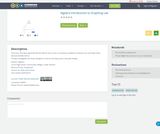Unrestricted Use
Public Domain
Rating

This lab is generally the first lab for this course. It introduces students to desmos.com and helps them become familiar with it. The lab investigates the visual changes to a line as the slope and y-intercept change.

Subject:
Mathematics
Algebra
Material Type:
Activity/Lab
Author:
Dr. Mary Alice Smeal
08/13/2020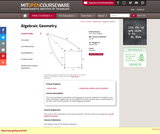Conditional Remix & Share Permitted
CC BY-NC-SA
Rating

" This course provides an introduction to the language of schemes, properties of morphisms, and sheaf cohomology. Together with 18.725 Algebraic Geometry, students gain an understanding of the basic notions and techniques of modern algebraic geometry."

Subject:
Algebra
Geometry
Material Type:
Full Course
Provider:
MIT
Provider Set:
MIT OpenCourseWare
Author:
Kedlaya, Kiran
01/01/2009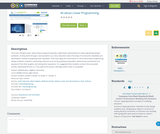Rating

Students learn about linear programming (also called linear optimization) to solve engineering design problems. As they work through a word problem as a class, they learn about the ideas of constraints, feasibility and optimization related to graphing linear equalities. Then they apply this information to solve two practice engineering design problems related to optimizing materials and cost by graphing inequalities, determining coordinates and equations from their graphs, and solving their equations. It is suggested that students conduct the associated activity, Optimizing Pencils in a Tray, before this lesson, although either order is acceptable.

Subject:
Mathematics
Algebra
Geometry
Material Type:
Lesson
Author:
Andi Vicksman
Malinda Zarske
Nathan Coyle
Russell Anderson
Ryan Sullivan
08/11/2020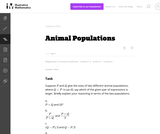Unrestricted Use
CC BY
Rating

In this task students have to interpret expressions involving two variables in the context of a real world situation. All given expressions can be interpreted as quantities that one might study when looking at two animal populations.

Subject:
Mathematics
Algebra
Material Type:
Activity/Lab
Provider:
Illustrative Mathematics
Provider Set:
Illustrative Mathematics
Author:
Illustrative Mathematics